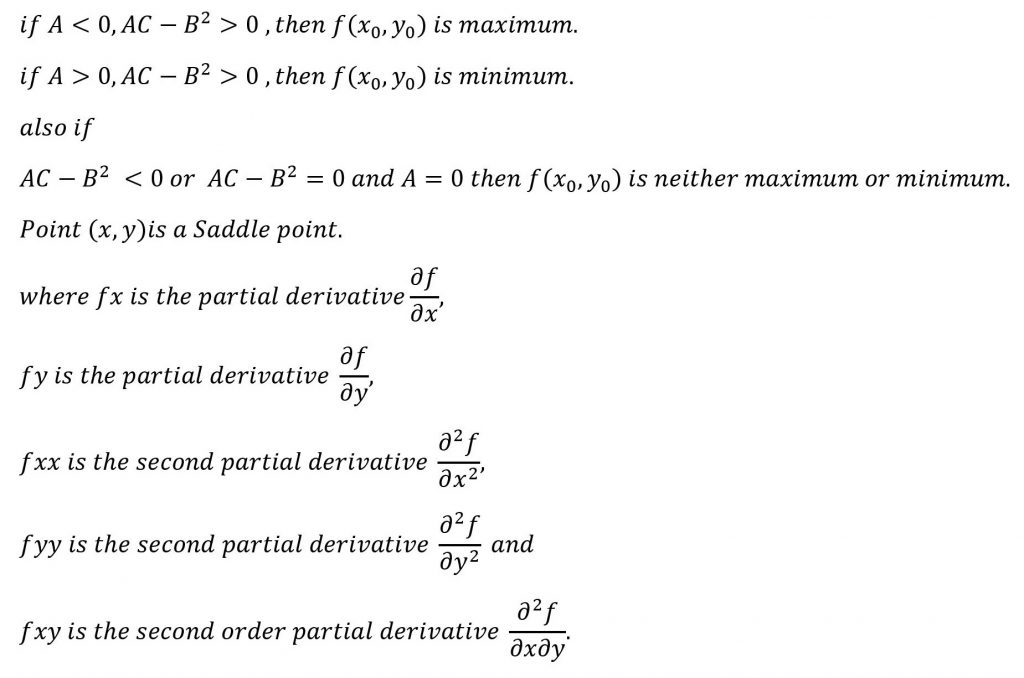## Maxima and Minima for a function of two variables

1. A function f(x) is said to have a maximum value at a point x=a if there exists a neibourhood of the point ‘a’ say (a+h), h is small, such that f(a)>f(a+h).

2. Similarly if f(a)<f(a+h) then f(x)is said to have minimum value at x=a.

3. A necessary condition for f(a) to be an extreme value of f(x) is that f'(a)=0
f(a)is maximum if f'(a)=0 and f”(a)<0
f(a)is minimum if f'(a)=0 and f”(a)>0

## Procedure

Step-1 : Find stationary points (x,y) such that fx=0 and fy=0.

Step-2 : Find A=fxx, B=fxy, C=fyy.

Step-3 : At every stationary point, find AC-B^2.